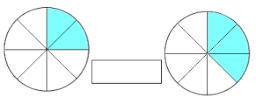# A quotient

What is the quotient of 3/10 divided by 2/4 as a fraction?

k =  0.6

### Step-by-step explanation:

$k=\frac{3}{10}\mathrm{/}\frac{2}{4}=\frac{3}{5}=0.6$Did you find an error or inaccuracy? Feel free to write us. Thank you!

Showing 1 comment:Math student
y'all are the bestTips to related online calculators
Need help to calculate sum, simplify or multiply fractions? Try our fraction calculator.

## Related math problems and questions:

• Quotient and divisionFind the quotient of 3/4 and 1/4.
• FractionsSort fractions z1 = (6)/(11); z2 = (10)/(21); z3 = (19)/(22) by its size. Result write as three serial numbers 1,2,3.
• Evaluate mixed expressionsWhich of the following is equal to 4 and 2 over 3 divided by 3 and 1 over 2? A. 4 and 2 over 3 times 3 and 2 over 1 B. 14 over 3 times 2 over 7 C. 14 over 3 times 7 over 2 D. 42 over 3 times 2 over 31
• Quotient 3If the quotient of 8/13 and 2 is subtracted from the product of 1 3/4 and 8/21, what is the difference?
• What is 11What is the quotient of Three-fifths and 1 Over 10?
• Fraction to decimalWrite the fraction 3/22 as a decimal.
• Numbers divisionWith what number should be divided mixed number 2 3/4 to get 11/12?Why does 1 3/4 + 2 9/10 equal 4.65? How do you solve this?What is the sum of 2/3 and 3/10?Add two mixed fractions: 2 4/6 + 1 3/6
• Evaluate expr with 2 varsIf a=6 and x=2, find the value of (2ax + 7x- 10) /(4ax-3a-2)
• Fractions 4How many 2/3s are in 6?
• Fraction + eqSolve following simple equation with fractions: -5/6(8+5b) = 75 + 5/3b
• Sum of fractionsWhat is the sum of 2/3+3/5?
• Quotient and productIf the quotient of [8/5 divided by 8/10] is added to the product of [8/14 x 7/12 x 3/8], what is the sum?
• Evaluate 11Multiply the quotient of 6 and 2 by 3, then add 1. Add 1 and 6, then divide by 2 and multiply by 3. Divide 6 by the product of 2 and 3, then add 1.
• Mixed divided with fracThere are 2 2/3 pizzas. How many people are sharing when each has 2/3 of pizza?